Next: Photoelectric Effect Up: Wave-Particle Duality Previous: Representation of Waves via

# Classical Light Waves

Consider a classical, monochromatic, linearly polarized, plane light wave, propagating through a vacuum in the-direction. It is convenient to characterize a light wave (which is, of course, a type of electromagnetic wave) by specifying its associated electric field. Suppose that the wave is polarized such that this electric field oscillates in the-direction. (According to standard electromagnetic theory, the magnetic field oscillates in the-direction, in phase with the electric field, with an amplitude which is that of the electric field divided by the velocity of light in vacuum.) Now, the electric field can be conveniently represented in terms of a complex wavefunction: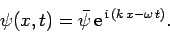(46)

Here,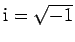,andare real parameters, and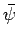is a complex wave amplitude. By convention, the physical electric field is the real part of the above expression. Suppose that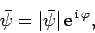(47)

where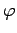is real. It follows that the physical electric field takes the form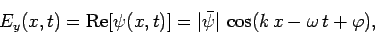(48)

where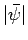is the amplitude of the electric oscillation,the wavenumber,the angular frequency, andthe phase angle. In addition,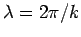is the wavelength, andthe frequency (in hertz).

According to standard electromagnetic theory, the frequency and wavelength of light waves are related according to the well-known expression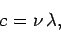(49)

or, equivalently,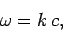(50)

where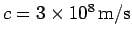. Equations (48) and (50) yield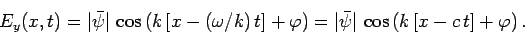(51)

Note that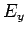depends onandonly via the combination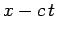. It follows that the wave maxima and minima satisfy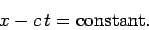(52)

Thus, the wave maxima and minima propagate in the-direction at the fixed velocity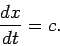(53)

An expression, such as (50), which determines the wave angular frequency as a function of the wavenumber, is generally termed a dispersion relation. As we have already seen, and as is apparent from Eq. (51), the maxima and minima of a plane wave propagate at the characteristic velocity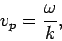(54)

which is known as the phase velocity. Hence, the dispersion relation (50) is effectively saying that the phase velocity of a plane light wave propagating through a vacuum always takes the fixed value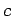, irrespective of its wavelength or frequency.

Now, from standard electromagnetic theory, the energy density (i.e., the energy per unit volume) of a light wave is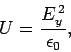(55)

where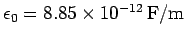is the permittivity of free space. Hence, it follows from Eqs. (46) and (48) that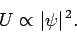(56)

Furthermore, a light wave possesses linear momentum, as well as energy. This momentum is directed along the wave's direction of propagation, and is of density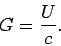(57)Next: Photoelectric Effect Up: Wave-Particle Duality Previous: Representation of Waves via
Richard Fitzpatrick 2010-07-20